Test: Fluid Pressure & Measurement

# Test: Fluid Pressure & Measurement

Test Description

## 10 Questions MCQ Test GATE Civil Engineering (CE) 2023 Mock Test Series | Test: Fluid Pressure & Measurement

Test: Fluid Pressure & Measurement for Civil Engineering (CE) 2022 is part of GATE Civil Engineering (CE) 2023 Mock Test Series preparation. The Test: Fluid Pressure & Measurement questions and answers have been prepared according to the Civil Engineering (CE) exam syllabus.The Test: Fluid Pressure & Measurement MCQs are made for Civil Engineering (CE) 2022 Exam. Find important definitions, questions, notes, meanings, examples, exercises, MCQs and online tests for Test: Fluid Pressure & Measurement below.
Solutions of Test: Fluid Pressure & Measurement questions in English are available as part of our GATE Civil Engineering (CE) 2023 Mock Test Series for Civil Engineering (CE) & Test: Fluid Pressure & Measurement solutions in Hindi for GATE Civil Engineering (CE) 2023 Mock Test Series course. Download more important topics, notes, lectures and mock test series for Civil Engineering (CE) Exam by signing up for free. Attempt Test: Fluid Pressure & Measurement | 10 questions in 30 minutes | Mock test for Civil Engineering (CE) preparation | Free important questions MCQ to study GATE Civil Engineering (CE) 2023 Mock Test Series for Civil Engineering (CE) Exam | Download free PDF with solutions
 1 Crore+ students have signed up on EduRev. Have you?
Test: Fluid Pressure & Measurement - Question 1

### The piezometric head in a static liquid

Test: Fluid Pressure & Measurement - Question 2

### In a mercury differential manometer used for measuring pressure differences across a venturimeter in a water pipeline, if an error of 2 mm has been made in observing a differential head of 10 mm, the percentage error in pressure difference is:

Detailed Solution for Test: Fluid Pressure & Measurement - Question 2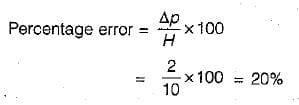Test: Fluid Pressure & Measurement - Question 3

### What is the pressure in Pascals at a depth of 1m below the water surface?

Detailed Solution for Test: Fluid Pressure & Measurement - Question 3

It’s the summation of weights on top of the water surface. In this case, it is the weight of the atmosphere and water above 1m. [Formula: P (depth) = Patm + (density of water*gravitational constant * depth)].

Test: Fluid Pressure & Measurement - Question 4

The left leg of a U-tube mercury manometer is connected to a pipeline conveying water, the level of mercury in the leg being 0.6 m below the centre of pipeline, and the right leg is open to atmosphere. The level of mercury in the right leg is 0.45 m above that in the left leg and the space above mercury in the right leg contains Benzene (specific gravity 0.88) to a height of 0.3 m. Find the pressure in the pipe in metres of water.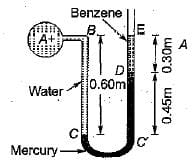Detailed Solution for Test: Fluid Pressure & Measurement - Question 4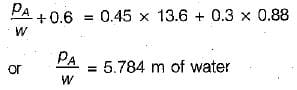Test: Fluid Pressure & Measurement - Question 5

The pressure, in meters of oil (sp. gr, 0,8), equivalent to 80 m of water is

Detailed Solution for Test: Fluid Pressure & Measurement - Question 5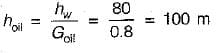Test: Fluid Pressure & Measurement - Question 6

Match List-1 (Features) with List-ll .(Type of manometers) and select the correct answer using the codes given below the lists:
List-I
A. Open ended manometer for positive pressure
B. Negative pressure manometer
C. For measuring pressure in liquids or gases
D. For measuring pressure in liquids only

List-ll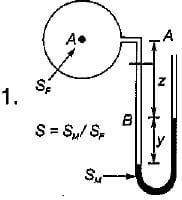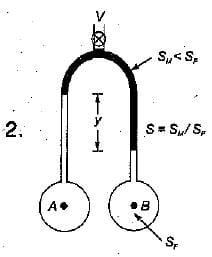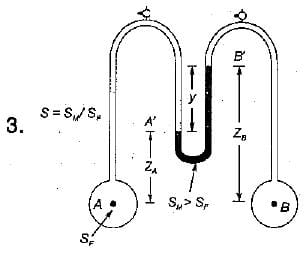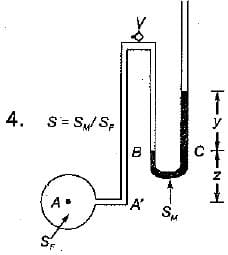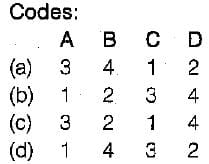Test: Fluid Pressure & Measurement - Question 7

Absolute pressure in flow system

Detailed Solution for Test: Fluid Pressure & Measurement - Question 7

The pressure measured above the absolute zero or complete vacuum is called absolute pressure.
Absolute pressure = Gauge pressure + Local atmosphere pressure.
also Absolute pressure = Local atmosphere pressure - Negative gauge pressure.

Test: Fluid Pressure & Measurement - Question 8

Pressure of 200 kPa is equivalent to a head of h metres of carbon tetrachloride of relative density 1.59 where h is equal to

Detailed Solution for Test: Fluid Pressure & Measurement - Question 8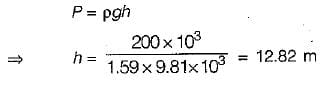Test: Fluid Pressure & Measurement - Question 9

Mercury is considered as a superior barometric fluid chiefly due to its

Detailed Solution for Test: Fluid Pressure & Measurement - Question 9

Mercury is considered as a superior barometric fluid because of following reasons:
1. High specific gravity- For a same pressure head mercury tube length required will be smaller than that of water
2.Low Vapour Pressure: Thus, influence in readings due to mercury vapours is negligible.

Test: Fluid Pressure & Measurement - Question 10

If a Mohr's circle is drawn for a fluid element inside a fluid body at rest, it would be

## GATE Civil Engineering (CE) 2023 Mock Test Series

25 docs|282 tests
 Use Code STAYHOME200 and get INR 200 additional OFF Use Coupon Code
Information about Test: Fluid Pressure & Measurement Page
In this test you can find the Exam questions for Test: Fluid Pressure & Measurement solved & explained in the simplest way possible. Besides giving Questions and answers for Test: Fluid Pressure & Measurement, EduRev gives you an ample number of Online tests for practice

## GATE Civil Engineering (CE) 2023 Mock Test Series

25 docs|282 tests